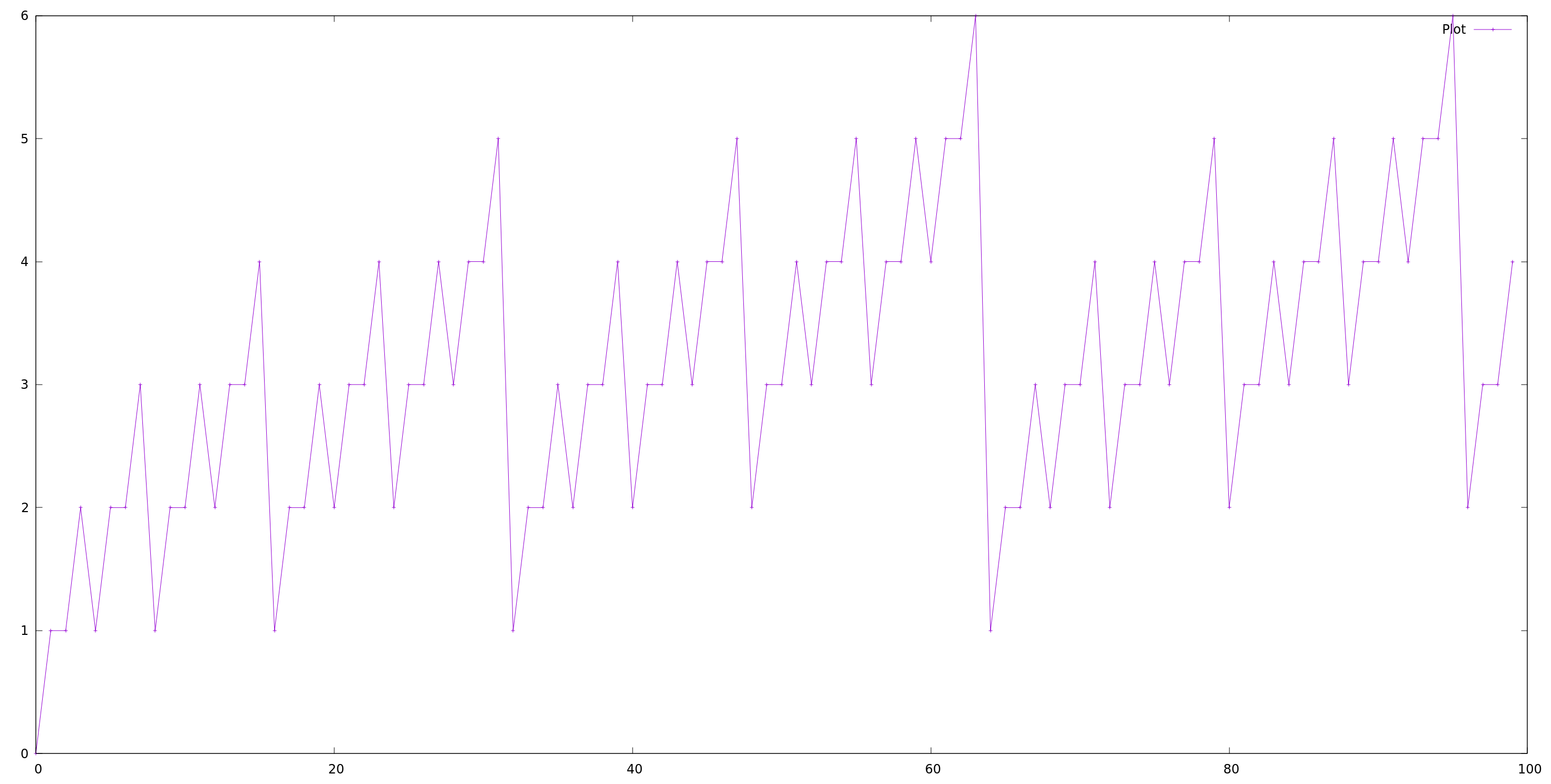# Challenge RE # 4

## Introduction

This is my solution to challenge 4. The assembly code to understand is the following:

``````<f>:
0:          mov    edx,edi
2:          shr    edx,1
4:          and    edx,0x55555555
a:          sub    edi,edx
c:          mov    eax,edi
e:          shr    edi,0x2
11:          and    eax,0x33333333
16:          and    edi,0x33333333
1e:          mov    eax,edi
20:          shr    eax,0x4
25:          and    eax,0xf0f0f0f
2a:          imul   eax,eax,0x1010101
30:          shr    eax,0x18
33:          ret
``````

## Note

I tried to describe my whole thought process here, so at some point I describe ideas that didn’t lead to an answer. For example, the plotting of the results. If you are hurry you can skip that.

## Analisis

Let’s notice here that there are 4 constants that are quite intriguing at first, I’m sure this will be the first thing to note. A couple of questions arrive to your mind when you see those constants:

1. What are they for?
2. Why are so specific?
3. Is there a pattern in their binary representation?

Let’s start with the last one, that is the easiest one at the moment. Their binary representation is as follows:

``````
0x55555555 => 0b1010101010101010101010101010101
0x33333333 => 0b110011001100110011001100110011
0xf0f0f0f  => 0b1111000011110000111100001111
0x1010101  => 0b1000000010000000100000001

``````

You can check them yourself with bin in Python.

The first one,`0x55555555`, has alternated 1s and 0s, and has 31 bits. The second one, `0x33333333`, has alternated pairs of 1s and 0s, with 30 bits this time. Third one, `0xf0f0f0f`, has alternated sets of 4 ones and 4 zeros, with 28 bits. And the last one `0x1010101`, has ones in position 0, 8, 16 and 24 only, from left to right, with 25 bits.

Kind of weird, but we just started, so it’s normal to not understand everything at first. Let’s keep diging.

Let’s translate(I’m not sure if this is the correct term for this 😁) the code into C code. Given that it’s a small amount of code, and I have no idea which algorithm is this. It’s a good idea to see what sequence we get with this, and if it makes any sense for us.

``````#include <stdio.h>
#include <limits.h>

unsigned int f(int a)
{
// shr    edx,1
// and    edx,0x55555555
// sub    edi,edx
// mov    eax,edi
unsigned int b = (a - ((a >> 1) & 0x55555555));

// shr    edi,0x2
// and    eax,0x33333333
// and    edi,0x33333333
// mov    eax,edi
b = ((b >> 2) &  0x33333333) + (b & 0x33333333);

// shr    eax,0x4
// and    eax,0xf0f0f0f
// imul   eax,eax,0x1010101
// shr    eax,0x18
b = (b + (b >> 4)) & 0xf0f0f0f;
b *= 0x1010101;

return b >> 0x18;
}

``````

The sequence that we get is not obvious at all, in this situation it’s very convenient to plot this numbers in a cartesian chart to see what we get. Let’s do that.

## Plot

To plot the results I’ll use gnuplot. I normally use Ubuntu as OS, and for this specific case the installation it’s straight forward.

``````sudo apt update
sudo apt install gnuplot
``````

Now this will install gnuplot as a program in our system, in order to use it in our code, will be necessary to `pipe` our data into `gnuplot`. You can read more about piping in these two blogs, Pipes I and Pipes II. For that I’ll make use of popen.

I’ll plot the result for `x = [0, 100)`, the code for that would be as follows:

``````#include <stdio.h>
#include <stdlib.h>

// ...
// previous code with implementation of f
// ...

int plot()
{
int size = 100;
unsigned int x[size], y[size];
int i = 0;
for (i = 0; i < size; i++) {
x[i] = i;
y[i] = f(i);
}

FILE *gnuplot = popen("gnuplot", "w");
if (!gnuplot) {
perror("popen");
return 1;
}

fprintf(gnuplot, "plot '-' u 1:2 t 'Plot' w lp\n");
for (int i = 0; i < size; ++i) {
fprintf(gnuplot,"%d %d\n", x[i], y[i]);
}
fprintf(gnuplot, "e\n");
fprintf(stdout, "Click Ctrl+d to quit...\n");
fflush(gnuplot);
getchar();

pclose(gnuplot);

return 0;
}

int main()
{
return plot();
}
``````

On the plotting we get a surprise, I still have no idea what is this? But has an stair form pattern. Let me show you:## Printing sequence

Nothing really 😥. What if we print the the binary representation of the input ? Well as a result we get something like this:

``````i f(i) bin(i)
0 0    0b0
1 1    0b1
2 1    0b10
3 2    0b11
4 1    0b100
5 2    0b101
6 2    0b110
7 3    0b111
8 1    0b1000
9 2    0b1001
``````

Nice!! This is basically counting the number of bits set on the input. For example, number 9 has 2 bits set, 8 one bit, etc…

So we have the behaviour, now in the challenge there’s a question that I don’t know the answer and it’s as follows:

`Some versions have the 0x1010101 constant, some do not. Why?`

My answer is…🤔…I have no idea 😎. To be honest I’m happy with what I got until now, maybe in the future I’ll comeback and try to figure out why in some arquitectures it’s necessary the multiplication of `0x1010101`.